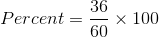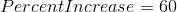# PSAT Math : How to find the percent of increase

## Example Questions

### Example Question #1 : How To Find The Percent Of Increase

The population of Town A is 12,979 people in 1995.  The population, when measured again in 2005, is 22,752.  What was the change in population to the nearest whole percentage point?

75%

175%

57%

68%

43%

75%

Explanation:

Since we are looking for the change, we must take the

(Ending Point – Starting Point)/Starting Point * 100%

(22752 – 12979)/12979 * 100%

9773/12979 * 100%

0.753 * 100%

75%

### Example Question #2 : How To Find The Percent Of Increase

A factory produced 2500 units during the month of September.  In order to increase production by 12% in the month of October, the factory hired more workers.  How many units were produced in October?

3600

3200

3000

4000

2800

2800

Explanation:

This is a percentage increase problem.

Easiest approach :  2500 x 1.12 = 2800

In this way you are adding 12% to the original.

Using the formula, find 12% of 2500

12/100 = x/2500,

30000 = 100x

300 = x

Now add that to the original to find the new production:

2500 + 300 = 2800

### Example Question #1 : How To Find The Percent Of Increase

The radius of a given circle is increased by 20%.  What is the percent increase of the area of the circle.

40%

20%

100%

144%

44%

44%

Explanation:

If we plug-in a radius of 5, then a 20% increase would give us a new radius of 6 (which is 1.2 x 5).  The area of the new circle is π(6)2 = 36π, and the area of the original circle was π(5)2 = 25π .  The numerical increase (or difference) is 36π - 25π = 11π.  Next we have to divide this difference by the original area: 11π/25π = .44, which multiplied by 100 gives us a percent increase of 44%.  The percent increase = (the numerical increase between the new and original values)/(original value) x 100. The algebraic solution gives us the same answer.  If radius r of a certain circle is increased by 20%, then the new radius would be (1.2)r.  The area of the new circle would be 1.44 π r2 and the area of the original circle πr2.  The difference between the areas is .44 π r2, which divided by the original area, π r2, would give us a percent increase of .44 x 100 = 44%.

### Example Question #3 : Percent Of Change

Phoenicia is a grocery store that is expanding quickly.

In 2011 Phoenicia's total sales were $1,800,800. In 2012 their sales rose to$2,130,346.

By what percentage did the store increase its income from 2011 to 2012.

(Round answer to the nearest tenth.)

16.4%

10.5%

19.2%

21.0%

18.3%

18.3%

Explanation:

$1,800,800 divided by 100 equals 18,008 and$2,130,346 divided by 18,008 is 118.3

So we know that \$2,130,346 is 118.3% of the sales in the previous year. Hence sales increased by 18.3%.

### Example Question #1 : How To Find The Percent Of Increase

Cindy is running for student body president and is making circular pins for her campaign. She enlarges her campaign image to fit the entire surface of a circular pin. After the image is enlarged, its new diameter is 75 percent larger than the original. By approximately what percentage has the area of the image increased?

75%

20%

100%

225%

200%

200%

Explanation:

Pick any number to be the original diameter. 10 is easy to work with. If the diameter is 10, the radius is 5. The area of the original image is A = πr2, so the original area = 25π. Now we increase the diameter by 75%, so the new diameter is 17.5. The radius is then 8.75. The area of the enlarged image is approximately 77π. To find the percentage by which the area has increased, take the difference in areas divided by the original area.  (77π - 25π)/25π = 51π/25π = 51/25 = 2.04 or approximately 200%

### Example Question #2 : How To Find The Percent Of Increase

If the side of a square is doubled in length, what is the percentage increase in area?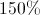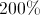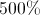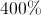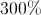Explanation:

The area of a square is given by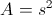, and if the side is doubled, the new area becomes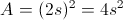.  The increase is a factor of 4, which is 400%.

### Example Question #1 : How To Find The Percent Of Increase

A rectangle has a width of 6 and a length of 10. If both the width and length are increased by 2, what is the percent increase of the area of the rectangle?

50%

160%

37.5%

60%

36%

60%

Explanation:

The equation to use for percent increase or decrease is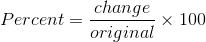to find the change in area, let's first calculate the area of the original. The formula for area of a rectangle is area=length x width. The original area, therefore, is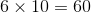The new rectangle has side lengths which are increased by 2, so the new lengths are 8 and 12. The area of the new rectangle, then is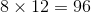.

The change in area is found by subtracting the the old area from the new area, so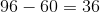When you input these numbers into the percent increase equation, you get